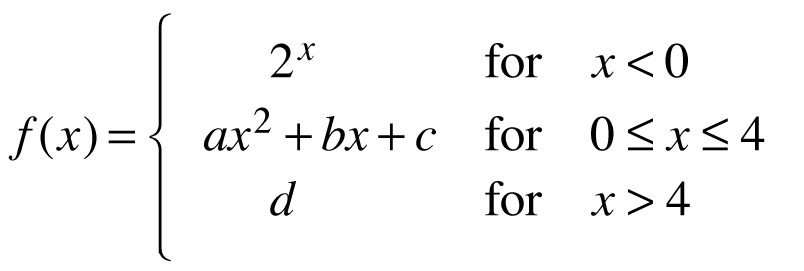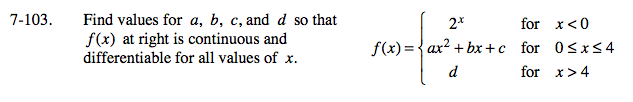### Home > CALC > Chapter 7 > Lesson 7.2.4 > Problem7-103

7-103.
1. Find values for a, b, c, and d so that f(x) below is continuous and differentiable for all values of x. Homework Help ✎Continuous implies that the pieces of the function agree at the boundary points.
Differentiable implies that the pieces of the derivative agree at the boundary points.

For each boundary point, x = 0 and x = 4, write and solve a system of equations.
One equation connects f(x), the other connects f '(x).